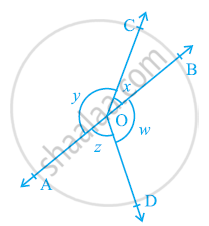# In the given figure, if x + y = w + z, then prove that AOB is a line - Mathematics

In the given figure, if x + y = w + z, then prove that AOB is a line.#### Solution

It can be observed that,

x + y + z + w = 360º            ....(Complete angle)

It is given that,

x + y = z + w

∴ x + y + x + y = 360º

2(x + y) = 360º

x + y = 180º

Since x and y form a linear pair, AOB is a line.

Concept: Pairs of Angles
Is there an error in this question or solution?

#### APPEARS IN

NCERT Class 9 Maths
Chapter 6 Lines and Angles
Exercise 6.1 | Q 4 | Page 97